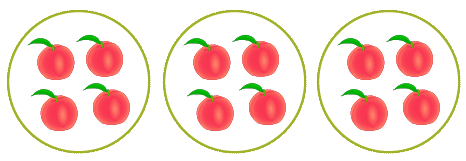# Write Repeated Addition And Multiplication For Equal Groups

## 'Repeated Addition And Multiplication Sentence For Equal Groups' Test and Printable Worksheets

A natural and excellent way to easily understand the multiplication concept is by introducing kids to the repeated addition method. In this concept however, we are going to write repeated addition and multiplication for equal groups.
Here, well designed models are presented to kids, making it very easy for them to identify and write both a repeated addition sentence as well as a multiplication sentence from just one model.

## Click the sections below to find more explanation on this topic

...

### Objective - multiplication sentences as repeated addition

The 'repeated addition and multiplication sentence for equal groups' test and printable worksheets will showcase to kids the relevance of the concept of equal groups in relation to addition and multiplication.

The product of multiplication can be obtained by combining several groups of equal sizes.

In a similar way, the same result can be obtained by repeatedly adding equal sizes of a given groups.
In the test however, kids are expected to find multiplication sentence and write repeated addition from models.

### Example: Describing multiplication sentence vs repeated addition for equal groups

We have 3 groups of apples and 4 apples per group, given the product as 12.Fill in the blank spaces describing the addition and multiplication sentences.

1. There are ___ groups of apples
2. ___ + ____ + ____ = 12
3. ____ x ____ = 12
• It is clear that there are 3 groups of apples.
• Next, We obtain our addition sentence by repeatedly adding sizes of apples in the three groups thus 4 + 4 + 4 = 12.
• Lastly, to obtain our multiplication sentence, we simply multiply the number of groups by the group size, I. e. 3 x 4 = 12.

Wow! Really awesome.
You can see that multiplication was related to addition.

I love this.